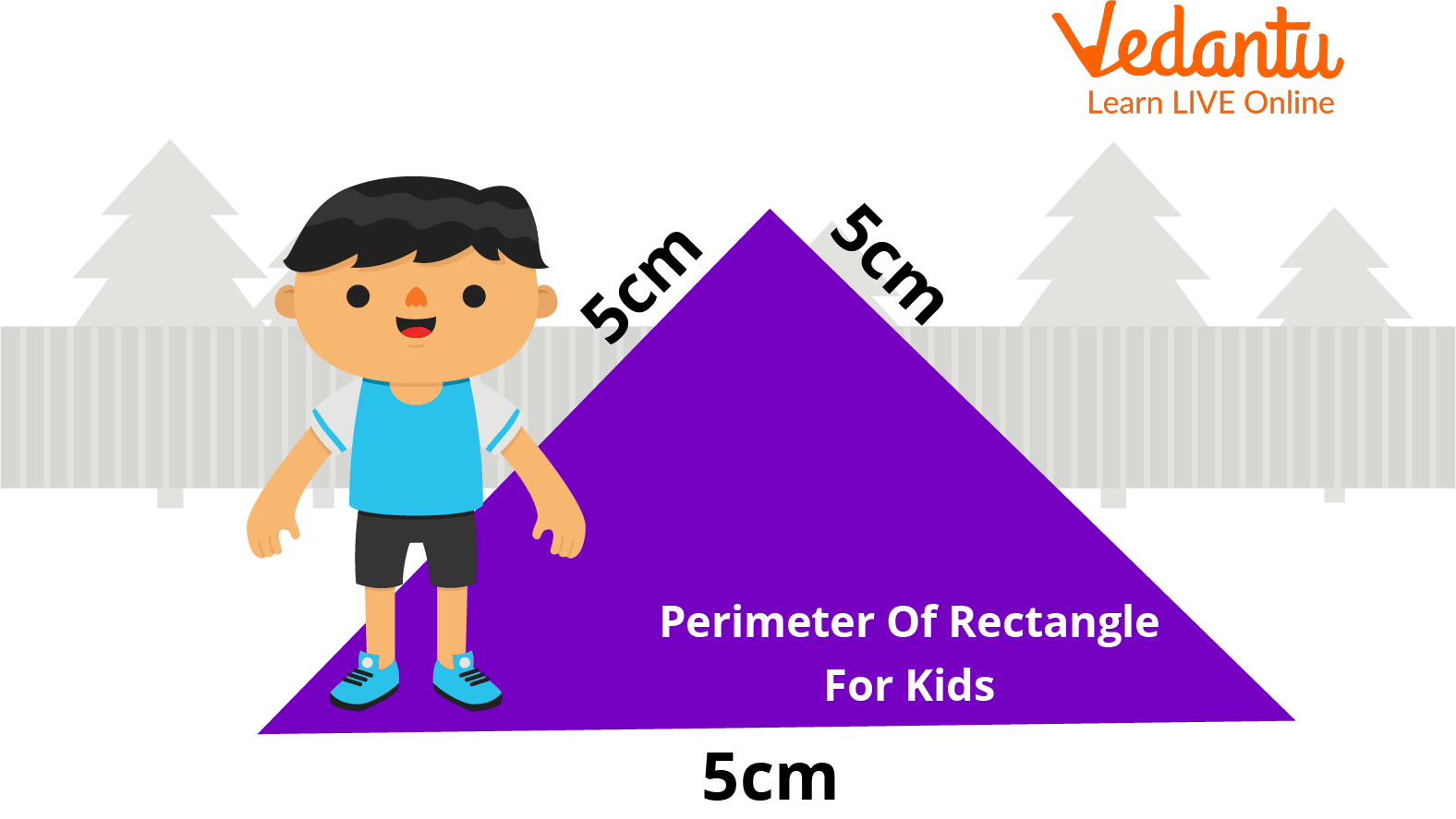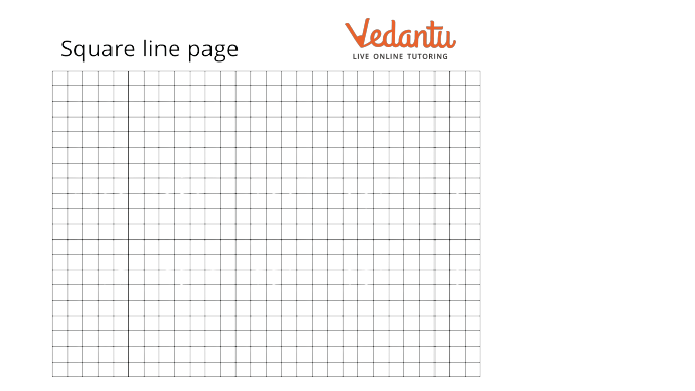Courses
Courses for Kids
Free study material
Offline Centres
More

# Perimeter ChartLast updated date: 30th Nov 2023
Total views: 99.3k
Views today: 2.99k## Introduction of Area and Perimeter

In mathematics, area and perimeter are the two important properties of any shape. It is used in our day-to-day lifestyle. The region enclosed by an object's shape is called the area. The shape's area can also be described as the area in a plane covered by a figure or any geometric shape. The dimensions and qualities of any shape determine its surface area.

Different shapes have different regions, each of which can be defined differently. The whole distance surrounding a figure is referred to as its perimeter. In a two-dimensional plane, a shape's perimeter is its entire circumference. Depending on their measurements, different shapes' perimeters may be equal in length.Perimeter

## Square Line Page:

It is a coordinate paper and grid paper. It is a type of writing paper printed with tiny lines forming a consistent grid. The lines are often used as a guide when producing graphs of functions or experimental data and sketching curves. It is frequently used in laboratory notebooks, as well as in mathematics and engineering education. Graph paper can be purchased either loose or bound in notebooks. Most graph paper pages have dimensions of 8.5 inches wide by 11 inches long.Square line page

## Different types of square line paper:

The different types of graph paper are:

• Grid Paper:- The widely used graph paper, on which students frequently plot or draw experimental data, curves, etc., is quite similar to grid paper. As the name implies, grid graph paper is mainly composed of regular grids, also known as standard lines in layman's terms.

• Quad Graph Paper:- This graph paper is referred to as the first kind of graph paper used, also known as quadrille graph paper. It is derived from French. The paper is grey and light blue in tone. Using a light grey hue, the grids are created. Usually made up of two to five blocks, quad graph paper displays smaller amounts of data.

• Engineering Graph Paper:- This graph paper is mainly for engineering-related tasks. The sheet of paper has ten grids and is bright green. Each page has a margin. This paper is mainly used to demonstrate a well-done job.

• Millimetre Graph Paper:- This graph paper is used for drawing tasks. Ten squares, each measuring one centimetre, make up the graph paper.

• Hexagonal Graph Paper:- The field of the geometric diagram uses this graph paper. The various structures are depicted on the graph.

• Dot Graph Paper:- Dot graph paper is simply another type of graph paper with a unique application among academics and professionals. Dot paper, frequently used for bullet journaling, drawing, etc., can be broadly defined as paper with dots spaced regularly across it.

### Conclusion:

A perimeter is a closed path that surrounds, encircles, or outlines a two-dimensional shape or a one-dimensional length. The perimeter is measured in unit. The perimeter chart of different figures is given below:

### Formulas

Square

4 × sides

Rectangle

2 × (length+width)

Triangle

Sides1+Sides2+Sides3

Circle

## FAQs on Perimeter Chart

1: Why should one learn about perimeter?

One of the most fundamental and important components of geometry, the perimeter, is most directly related to the characteristics of shapes, including 2D and 3D shapes. Before learning more complicated geometric concepts like area and volume, a kid must have a solid grasp on calculating a shape's perimeter.

2: How do we use perimeter in our daily life?

In daily life, we frequently use the idea of the perimeter. For instance, we measure the field's perimeter before constructing a fence around it to determine the length of wire we'll need.

3: Why is it called a perimeter?

A path that encloses a region is called a perimeter. The word is Greek, meaning "around," and "metre". The term can be considered the length of a shape's outline and can refer to either the path or its length. A circle's or an ellipse's circumference is known as its perimeter.